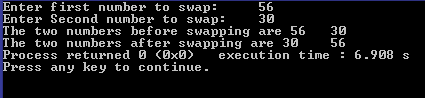Saturday, 27 December 2014

C Program to perform Swapping of 2 numbers

This is a C program which swaps the given 2 numbers . Here we use a temporary variable to perform swapping.

PROGRAM :
```#include <stdio.h>
#include <stdlib.h>
int main()
{
int number1,number2,num;
printf("Enter first number to swap:\t");
scanf("%d",&number1);
printf("Enter Second number to swap:\t");
scanf("%d",&number2);

printf("The two numbers before swapping are %d \t %d \n",
number1,number2);

num=number1;
number1=number2;
number2=num;

printf("The two numbers after swapping are %d \t %d",
number1,number2);

return 0;

}```
OUTPUT :C - Swapping of  2 numbers